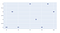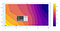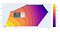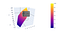# Easy XYZ Contouring using Plotly

By contouring, we can represent earth’s 3D landscape as a 2D map. It only takes 3 variables which are X, Y, and Z. Not only elevation data, we can create a 2D map of geospatial datasets from those 3 variables.

Most earth scientists and geographers rely on GIS to generate any kind of contouring-based map (GIS software is expensive, mostly). Contouring in Python usually requires extensive programming experience and It was an unpleasant experience for me (@_@). However I found a simple way to solve this unpleasant experience. Let’s start!

IMPORT

`#libraryimport numpy as npimport pandas as pdfrom numpy import linspace, meshgridfrom scipy.interpolate import griddataimport plotly.graph_objects as go`

After importing the required library , we should create a set of dummy data.

`#Dummy Datax = [2,3,4,6,7,8,9,10]y = [2,4,2,5,3,2,5,4]z = [100,80,90,70,70,60,40,40]`

Let’s take a look at the data

`fig = go.Figure()fig.update_layout(autosize=False)fig.add_Trace(go.Scatter(x=x, y=y, text=z, mode='markers+text'))`Scatter Plot of XYZ

From these data, we can directly create a 2D contouring map in Plotly

`fig = go.Figure()fig.add_trace(go.Contour(x=x,y=y,z=z,line_smoothing=1.3))fig.update_layout(autosize=False)fig.add_trace(go.Scatter(x=y,=y=y,text=z,mode='markers+text'))`You can hover your mouse pointer to get the value

It was a nice map. The next step we will try to use gridded value of Z by using `scipy.interpolate` to interpolate the z data.

`xi = linspace(min(x),max(x),200)yi = linspace(min(y),max(y),200)X,Y = meshgrid(xi,yi)Z = griddata((x,y),z,(X,Y), method='cubic')fig = go.Figure()fig.add_trace(go.Contour(z=Z, x=xi, y=yi, line_smoothing=1.3))fig.add_trace(go.Scatter(x=x,y=y, mode='markers+text', text=z))fig.update_layout(autosize=False)`Gridded Z Data

the advantage of gridded data is that we can get the interpolated XY coordinate when hovering the mouse pointer.

BONUS

`fig = go.Figure()fig.add_trace(go.Surface(z=Z, x=xi, y=yi))fig.update_layout(autosize=False)`Surface Plot

Cheers!

Random writer — Geology, Data, Tech, and Music

## More from Yogi P

Random writer — Geology, Data, Tech, and Music